Скачать презентацию 1 D Motion We begin our

db3a008a6f06b13ccd024e25516b540d.ppt

• Количество слайдов: 28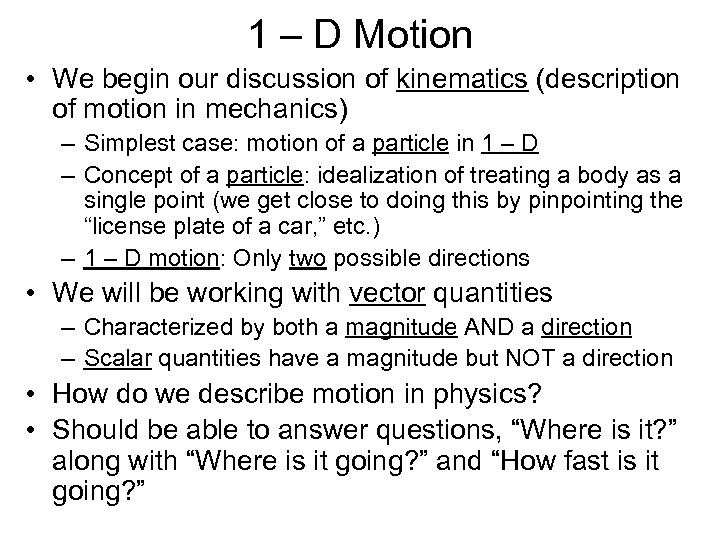1 – D Motion • We begin our discussion of kinematics (description of motion in mechanics) – Simplest case: motion of a particle in 1 – D – Concept of a particle: idealization of treating a body as a single point (we get close to doing this by pinpointing the “license plate of a car, ” etc. ) – 1 – D motion: Only two possible directions • We will be working with vector quantities – Characterized by both a magnitude AND a direction – Scalar quantities have a magnitude but NOT a direction • How do we describe motion in physics? • Should be able to answer questions, “Where is it? ” along with “Where is it going? ” and “How fast is it going? ”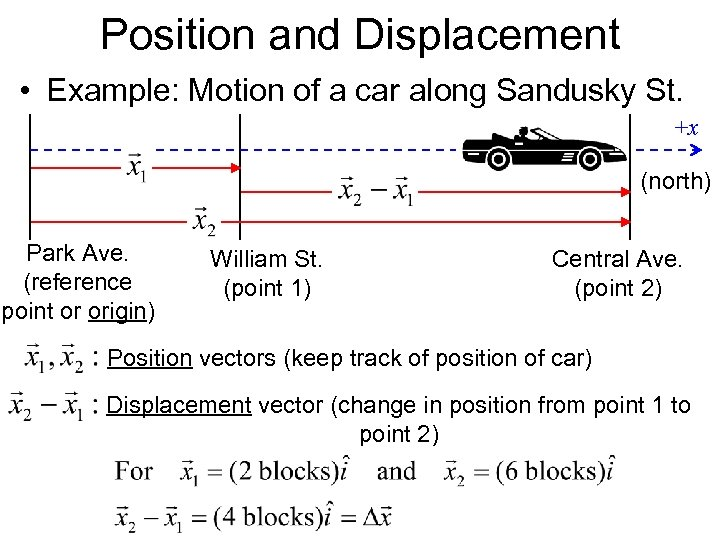Position and Displacement • Example: Motion of a car along Sandusky St. +x (north) Park Ave. (reference point or origin) William St. (point 1) Central Ave. (point 2) Position vectors (keep track of position of car) Displacement vector (change in position from point 1 to point 2)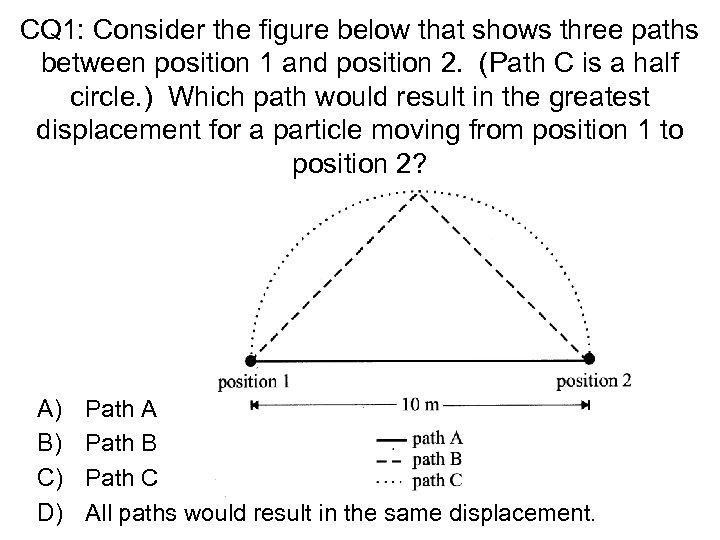CQ 1: Consider the figure below that shows three paths between position 1 and position 2. (Path C is a half circle. ) Which path would result in the greatest displacement for a particle moving from position 1 to position 2? A) B) C) D) Path A Path B Path C All paths would result in the same displacement.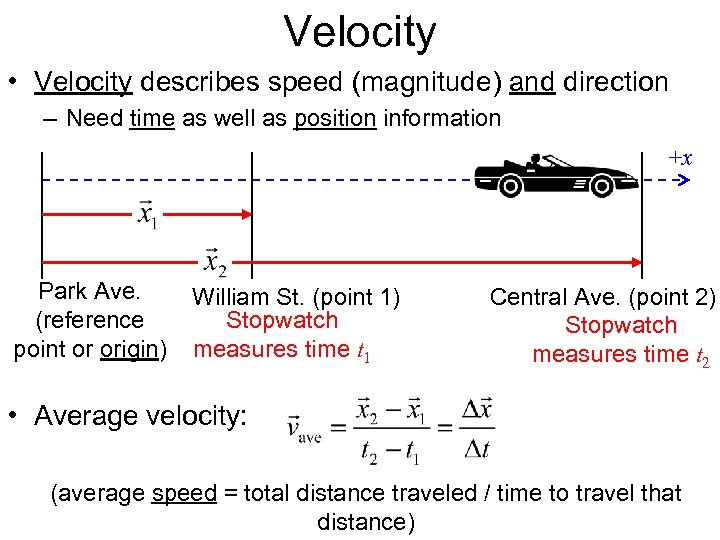Velocity • Velocity describes speed (magnitude) and direction – Need time as well as position information +x Park Ave. (reference point or origin) William St. (point 1) Stopwatch measures time t 1 Central Ave. (point 2) Stopwatch measures time t 2 • Average velocity: (average speed = total distance traveled / time to travel that distance)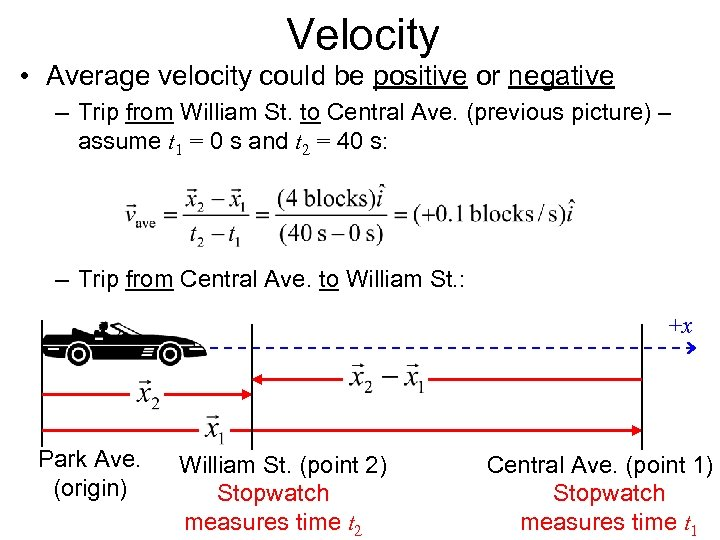Velocity • Average velocity could be positive or negative – Trip from William St. to Central Ave. (previous picture) – assume t 1 = 0 s and t 2 = 40 s: – Trip from Central Ave. to William St. : +x Park Ave. (origin) William St. (point 2) Stopwatch measures time t 2 Central Ave. (point 1) Stopwatch measures time t 1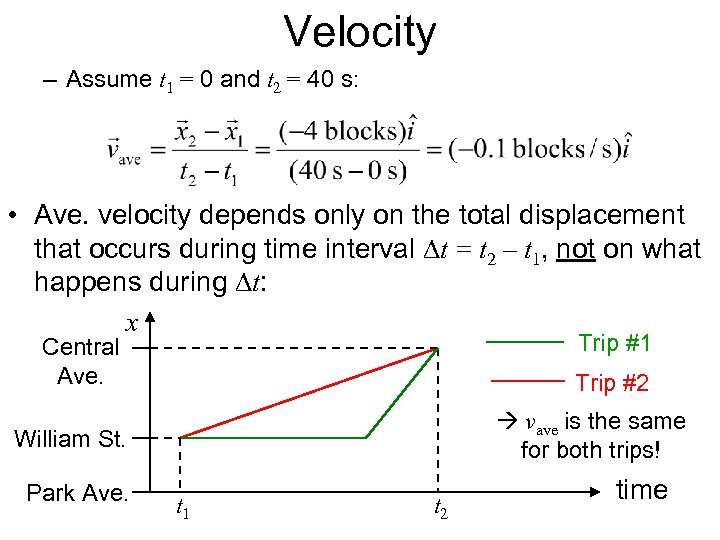Velocity – Assume t 1 = 0 and t 2 = 40 s: • Ave. velocity depends only on the total displacement that occurs during time interval Dt = t 2 – t 1, not on what happens during Dt: x Trip #1 Central Ave. Trip #2 vave is the same for both trips! William St. Park Ave. t 1 t 2 time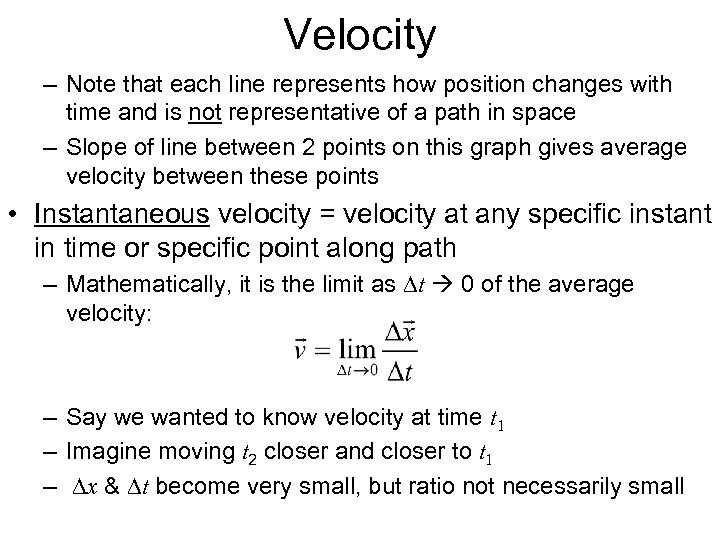Velocity – Note that each line represents how position changes with time and is not representative of a path in space – Slope of line between 2 points on this graph gives average velocity between these points • Instantaneous velocity = velocity at any specific instant in time or specific point along path – Mathematically, it is the limit as Dt 0 of the average velocity: – Say we wanted to know velocity at time t 1 – Imagine moving t 2 closer and closer to t 1 – Dx & Dt become very small, but ratio not necessarily small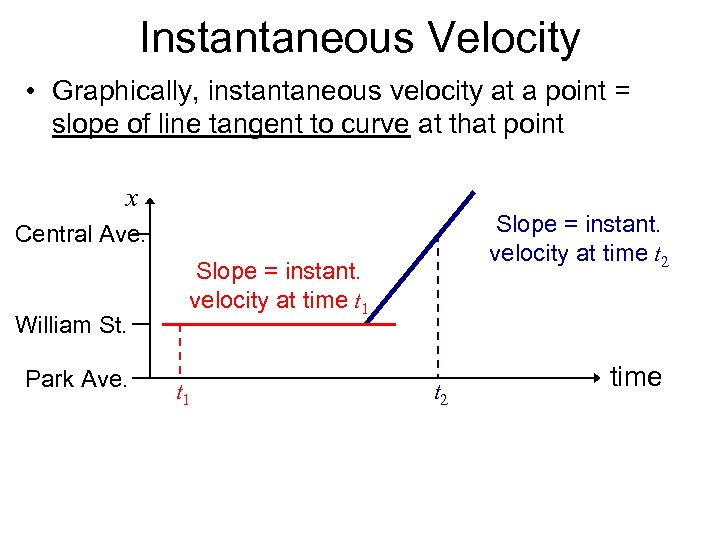Instantaneous Velocity • Graphically, instantaneous velocity at a point = slope of line tangent to curve at that point x Slope = instant. velocity at time t 2 Central Ave. William St. Park Ave. Slope = instant. velocity at time t 1 t 2 time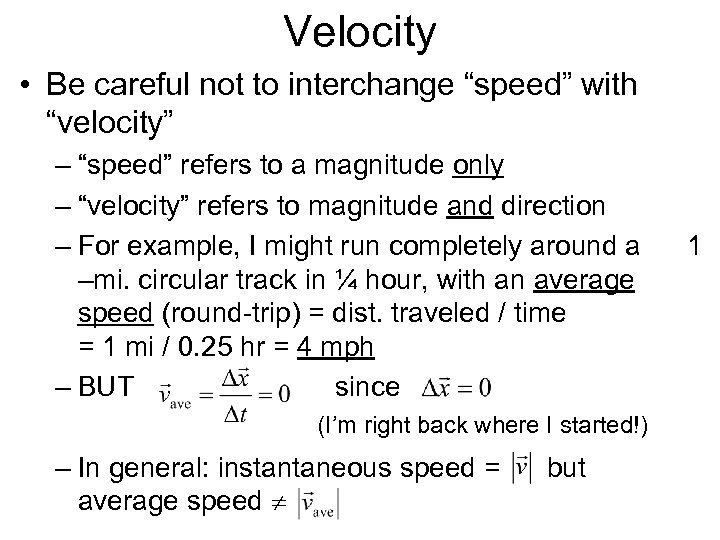Velocity • Be careful not to interchange “speed” with “velocity” – “speed” refers to a magnitude only – “velocity” refers to magnitude and direction – For example, I might run completely around a –mi. circular track in ¼ hour, with an average speed (round-trip) = dist. traveled / time = 1 mi / 0. 25 hr = 4 mph – BUT since (I’m right back where I started!) – In general: instantaneous speed = average speed but 1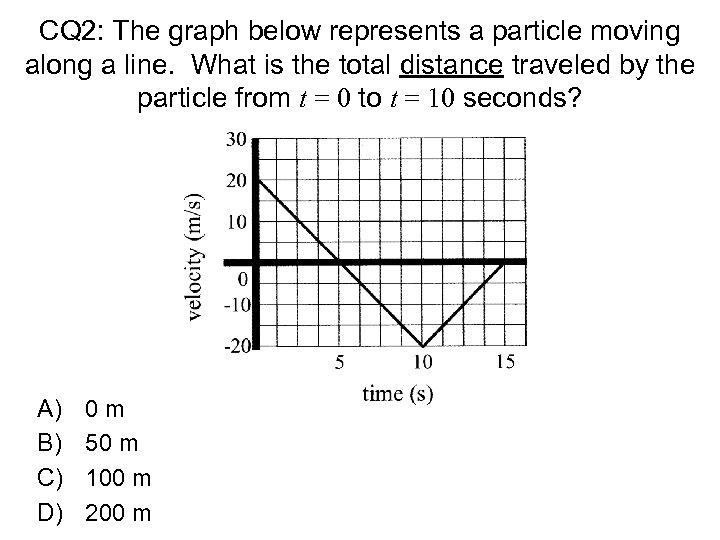CQ 2: The graph below represents a particle moving along a line. What is the total distance traveled by the particle from t = 0 to t = 10 seconds? A) B) C) D) 0 m 50 m 100 m 200 m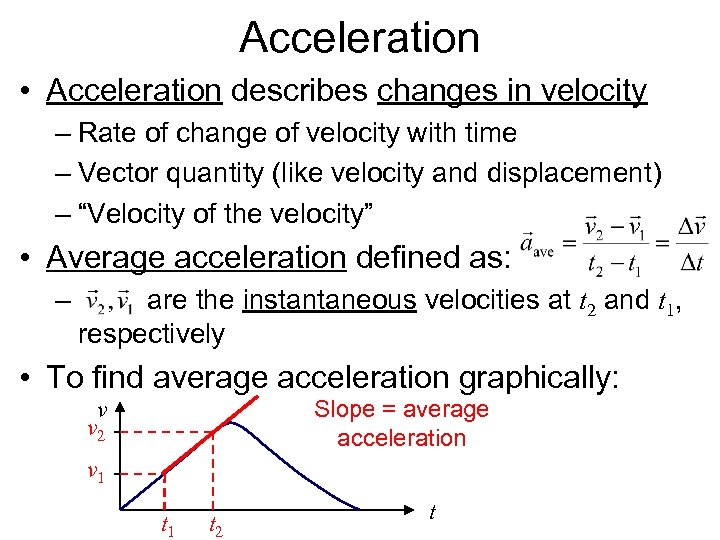Acceleration • Acceleration describes changes in velocity – Rate of change of velocity with time – Vector quantity (like velocity and displacement) – “Velocity of the velocity” • Average acceleration defined as: – are the instantaneous velocities at t 2 and t 1, respectively • To find average acceleration graphically: Slope = average acceleration v v 2 v 1 t 2 t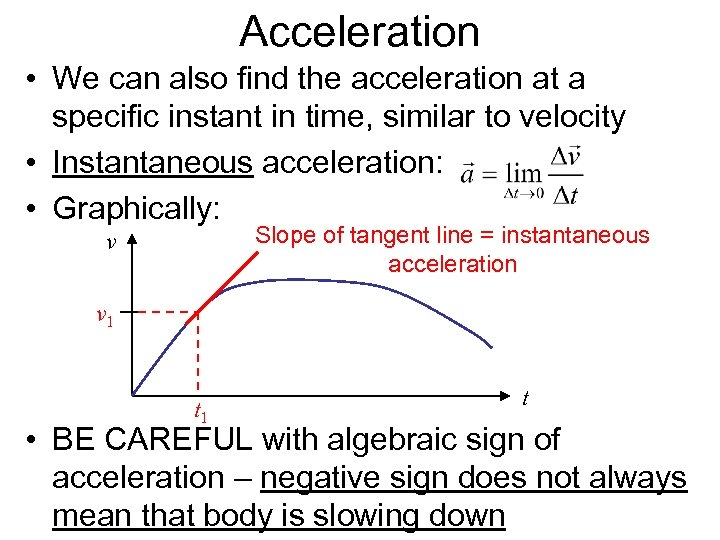Acceleration • We can also find the acceleration at a specific instant in time, similar to velocity • Instantaneous acceleration: • Graphically: Slope of tangent line = instantaneous acceleration v v 1 t • BE CAREFUL with algebraic sign of acceleration – negative sign does not always mean that body is slowing down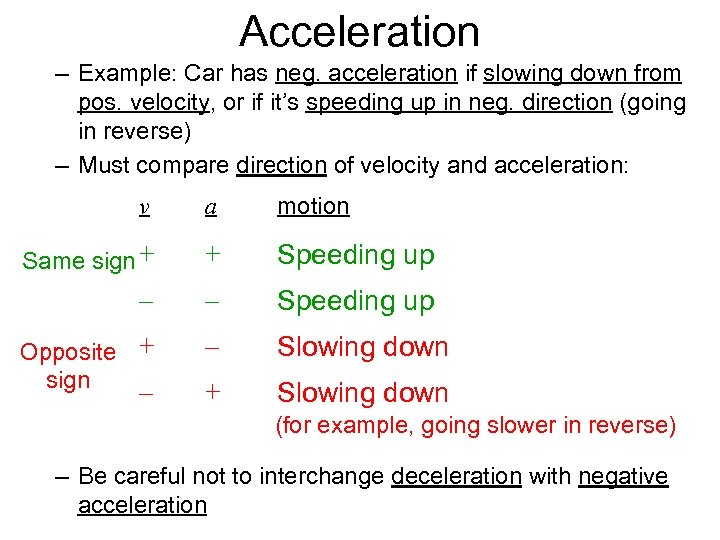Acceleration – Example: Car has neg. acceleration if slowing down from pos. velocity, or if it’s speeding up in neg. direction (going in reverse) – Must compare direction of velocity and acceleration: v a motion Same sign + + Speeding up – – Speeding up Opposite + sign – Slowing down + Slowing down – (for example, going slower in reverse) – Be careful not to interchange deceleration with negative acceleration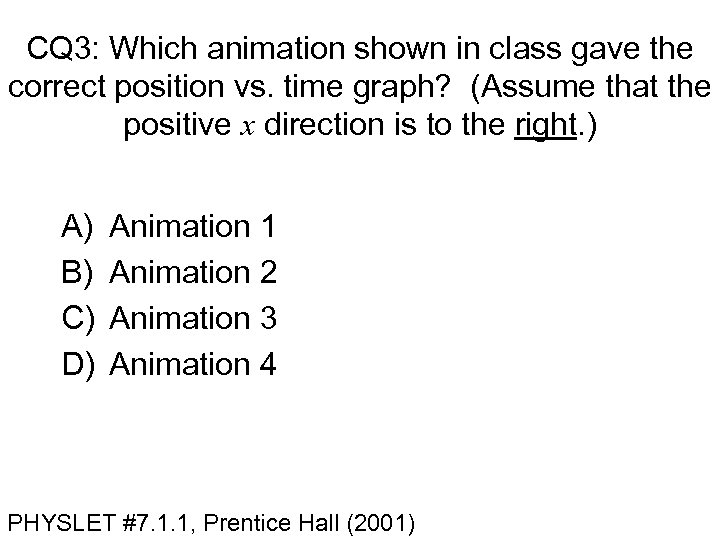CQ 3: Which animation shown in class gave the correct position vs. time graph? (Assume that the positive x direction is to the right. ) A) B) C) D) Animation 1 Animation 2 Animation 3 Animation 4 PHYSLET #7. 1. 1, Prentice Hall (2001)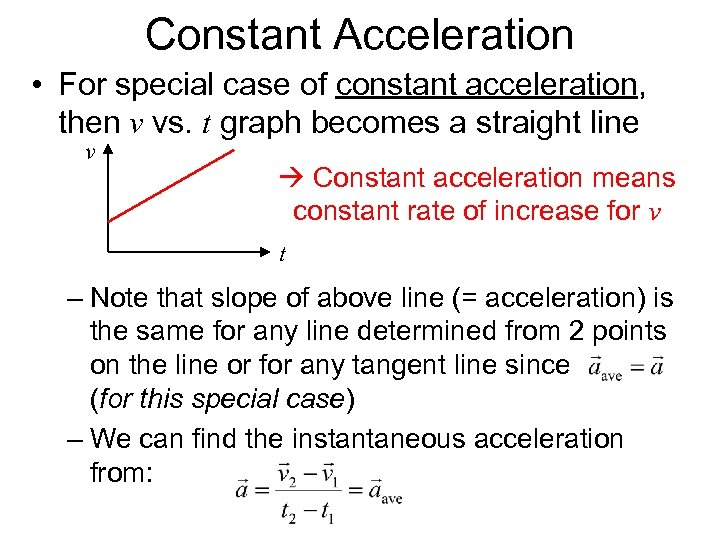Constant Acceleration • For special case of constant acceleration, then v vs. t graph becomes a straight line v Constant acceleration means constant rate of increase for v t – Note that slope of above line (= acceleration) is the same for any line determined from 2 points on the line or for any tangent line since (for this special case) – We can find the instantaneous acceleration from: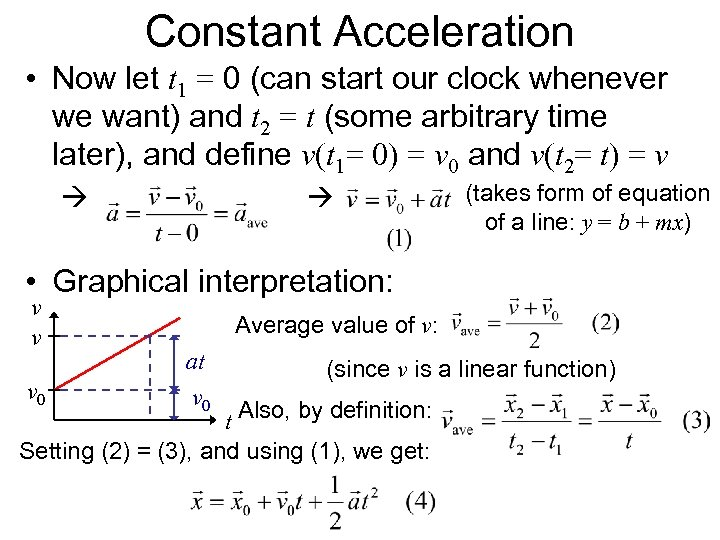Constant Acceleration • Now let t 1 = 0 (can start our clock whenever we want) and t 2 = t (some arbitrary time later), and define v(t 1= 0) = v 0 and v(t 2= t) = v (takes form of equation of a line: y = b + mx) • Graphical interpretation: v v v 0 Average value of v: at v 0 (since v is a linear function) t Also, by definition: Setting (2) = (3), and using (1), we get: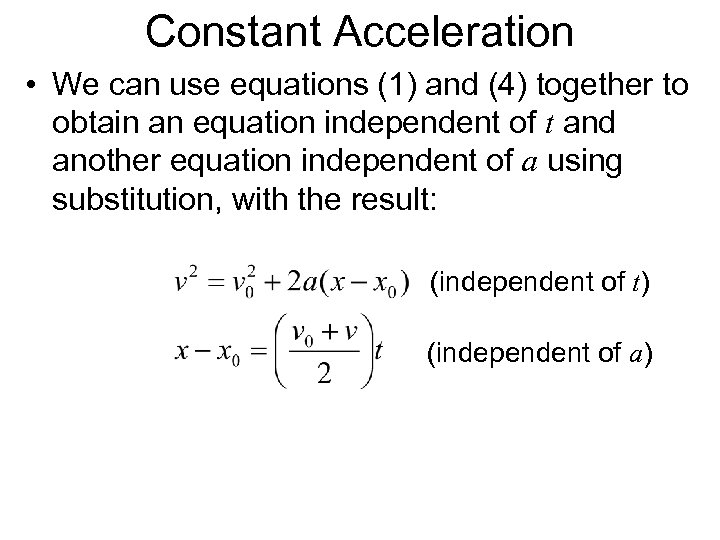Constant Acceleration • We can use equations (1) and (4) together to obtain an equation independent of t and another equation independent of a using substitution, with the result: (independent of t) (independent of a)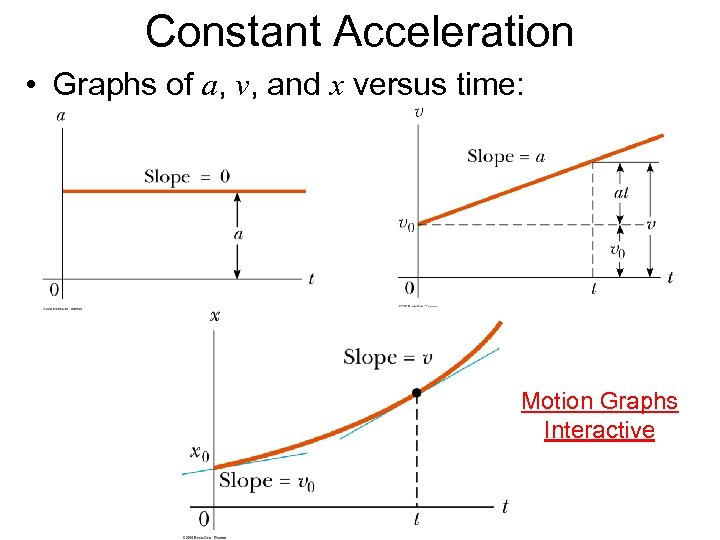Constant Acceleration • Graphs of a, v, and x versus time: Motion Graphs Interactive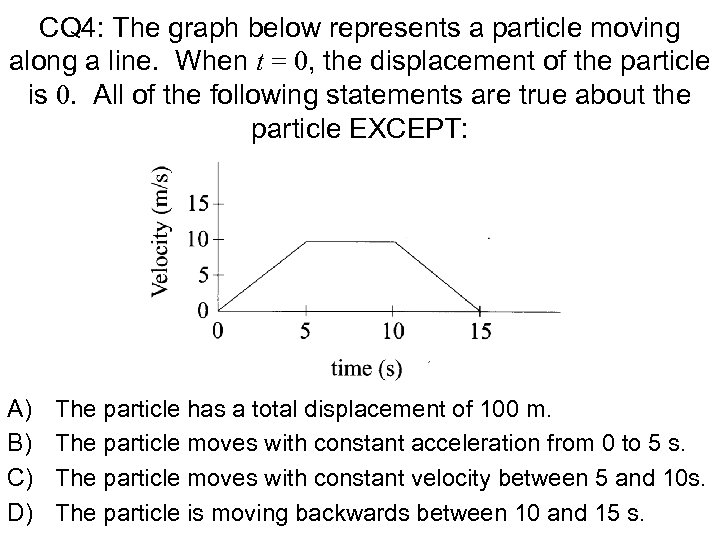CQ 4: The graph below represents a particle moving along a line. When t = 0, the displacement of the particle is 0. All of the following statements are true about the particle EXCEPT: A) B) C) D) The particle has a total displacement of 100 m. The particle moves with constant acceleration from 0 to 5 s. The particle moves with constant velocity between 5 and 10 s. The particle is moving backwards between 10 and 15 s.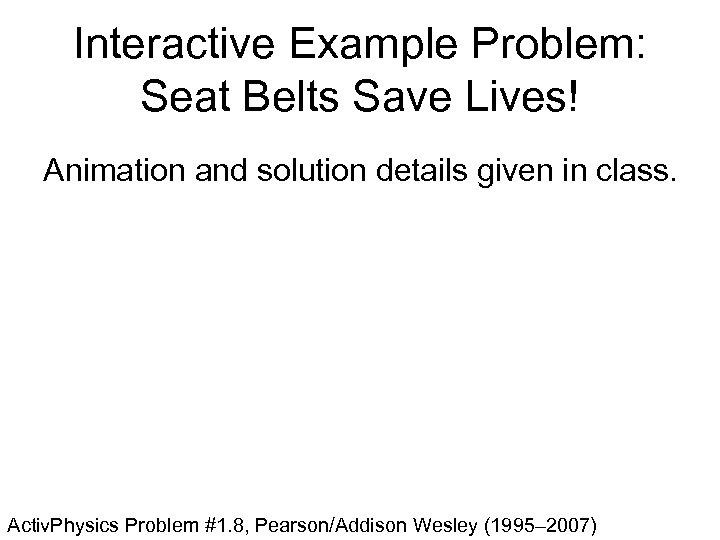Interactive Example Problem: Seat Belts Save Lives! Animation and solution details given in class. Activ. Physics Problem #1. 8, Pearson/Addison Wesley (1995– 2007)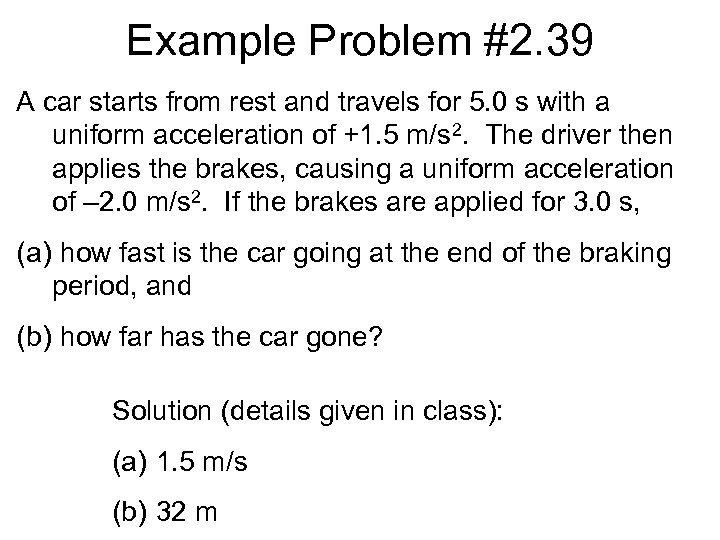Example Problem #2. 39 A car starts from rest and travels for 5. 0 s with a uniform acceleration of +1. 5 m/s 2. The driver then applies the brakes, causing a uniform acceleration of – 2. 0 m/s 2. If the brakes are applied for 3. 0 s, (a) how fast is the car going at the end of the braking period, and (b) how far has the car gone? Solution (details given in class): (a) 1. 5 m/s (b) 32 m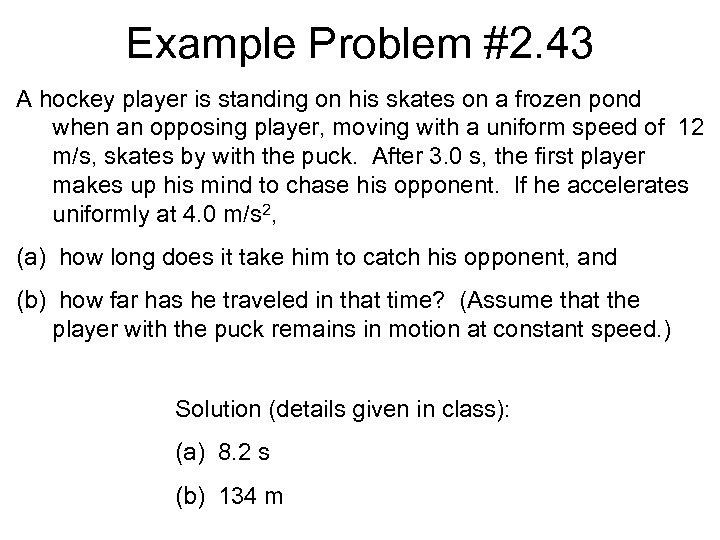Example Problem #2. 43 A hockey player is standing on his skates on a frozen pond when an opposing player, moving with a uniform speed of 12 m/s, skates by with the puck. After 3. 0 s, the first player makes up his mind to chase his opponent. If he accelerates uniformly at 4. 0 m/s 2, (a) how long does it take him to catch his opponent, and (b) how far has he traveled in that time? (Assume that the player with the puck remains in motion at constant speed. ) Solution (details given in class): (a) 8. 2 s (b) 134 m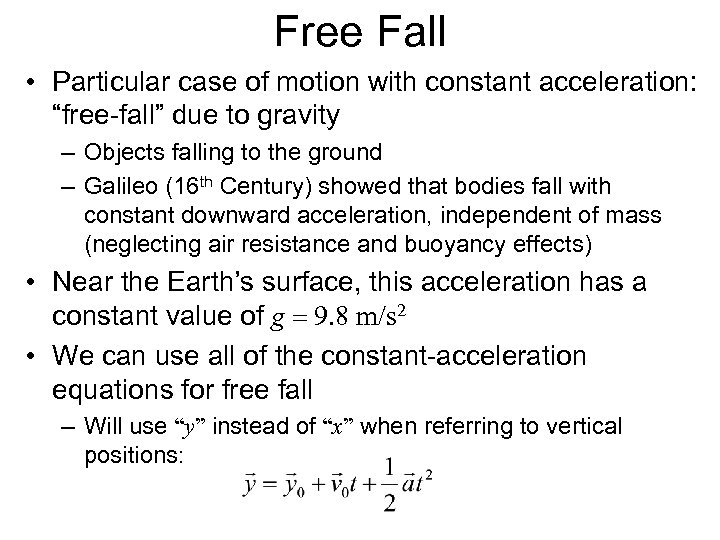Free Fall • Particular case of motion with constant acceleration: “free-fall” due to gravity – Objects falling to the ground – Galileo (16 th Century) showed that bodies fall with constant downward acceleration, independent of mass (neglecting air resistance and buoyancy effects) • Near the Earth’s surface, this acceleration has a constant value of g 9. 8 m/s 2 • We can use all of the constant-acceleration equations for free fall – Will use “y” instead of “x” when referring to vertical positions: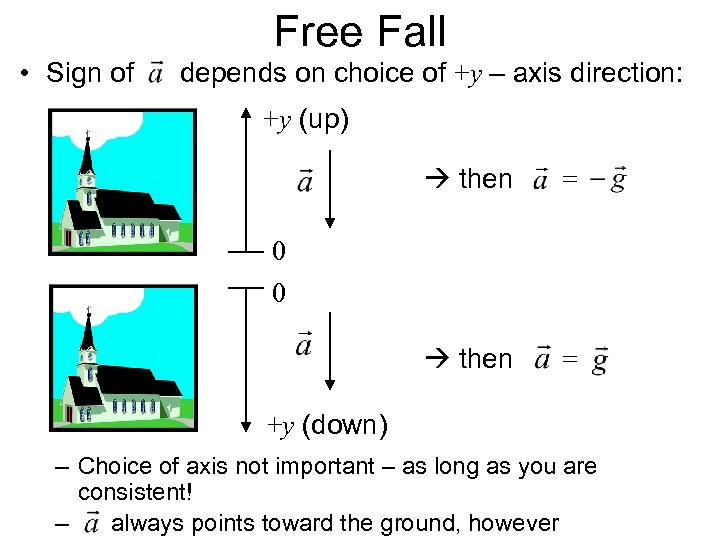Free Fall • Sign of depends on choice of +y – axis direction: +y (up) then = 0 0 +y (down) – Choice of axis not important – as long as you are consistent! – always points toward the ground, however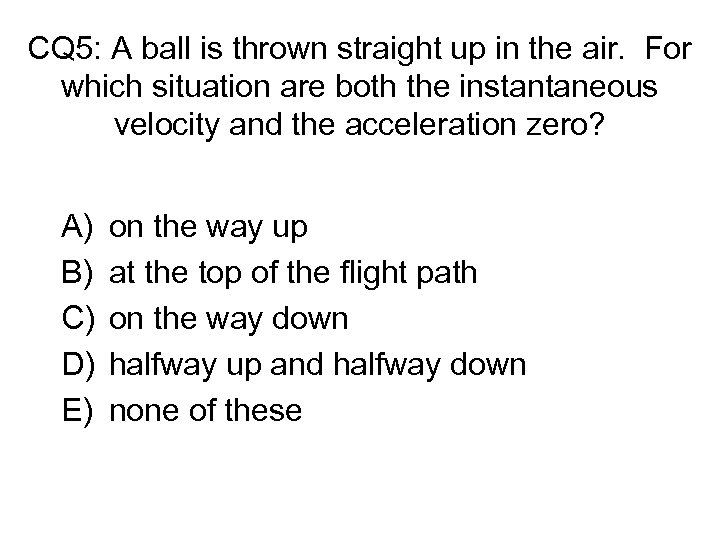CQ 5: A ball is thrown straight up in the air. For which situation are both the instantaneous velocity and the acceleration zero? A) B) C) D) E) on the way up at the top of the flight path on the way down halfway up and halfway down none of these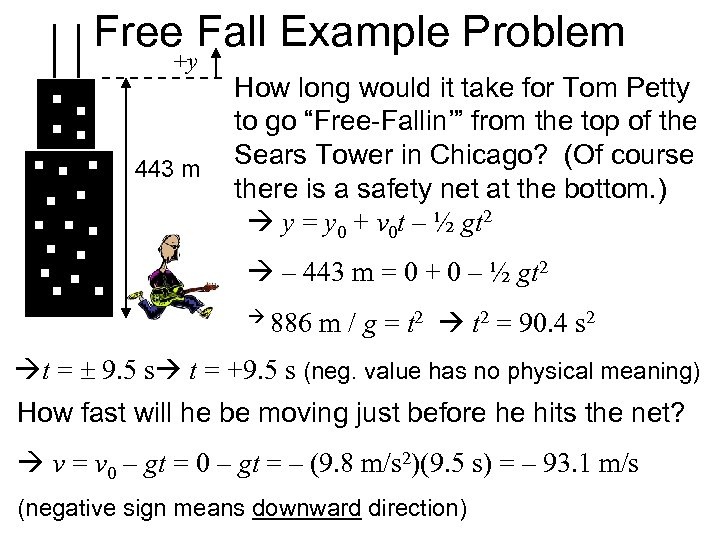Free Fall Example Problem +y 443 m How long would it take for Tom Petty to go “Free-Fallin’” from the top of the Sears Tower in Chicago? (Of course there is a safety net at the bottom. ) y = y 0 + v 0 t – ½ gt 2 – 443 m = 0 + 0 – ½ gt 2 886 m / g = t 2 = 90. 4 s 2 t = 9. 5 s t = +9. 5 s (neg. value has no physical meaning) How fast will he be moving just before he hits the net? v = v 0 – gt = – (9. 8 m/s 2)(9. 5 s) = – 93. 1 m/s (negative sign means downward direction)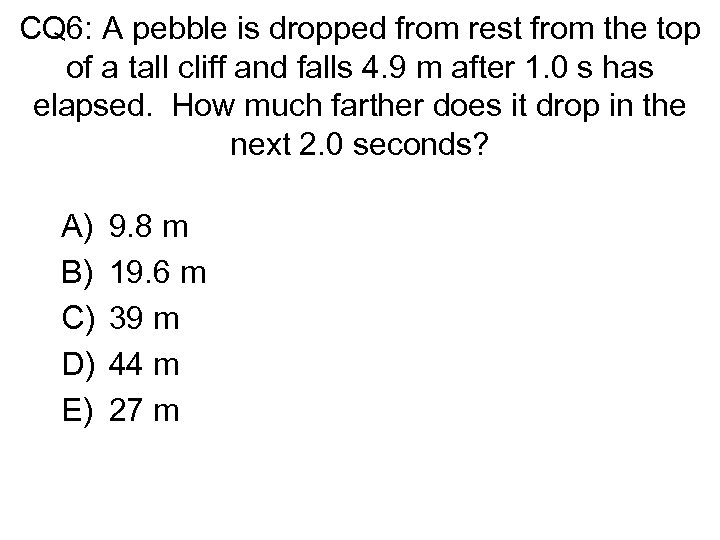CQ 6: A pebble is dropped from rest from the top of a tall cliff and falls 4. 9 m after 1. 0 s has elapsed. How much farther does it drop in the next 2. 0 seconds? A) B) C) D) E) 9. 8 m 19. 6 m 39 m 44 m 27 m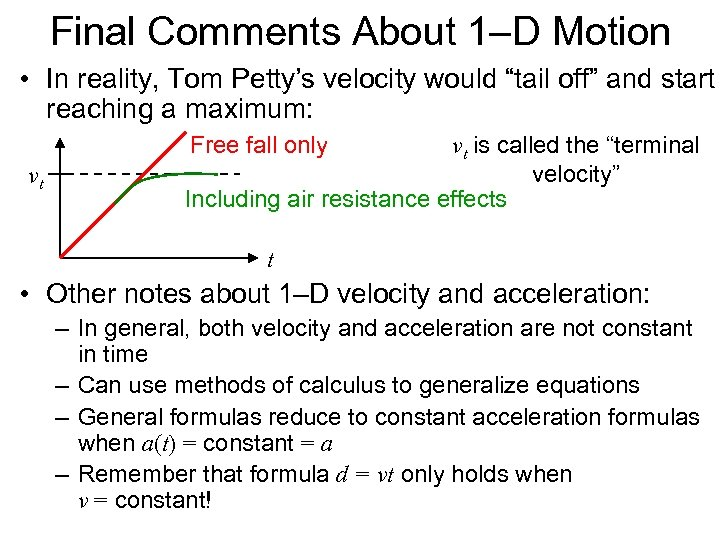Final Comments About 1–D Motion • In reality, Tom Petty’s velocity would “tail off” and start reaching a maximum: v vt vt is called the “terminal velocity” Including air resistance effects Free fall only t • Other notes about 1–D velocity and acceleration: – In general, both velocity and acceleration are not constant in time – Can use methods of calculus to generalize equations – General formulas reduce to constant acceleration formulas when a(t) = constant = a – Remember that formula d = vt only holds when v = constant!